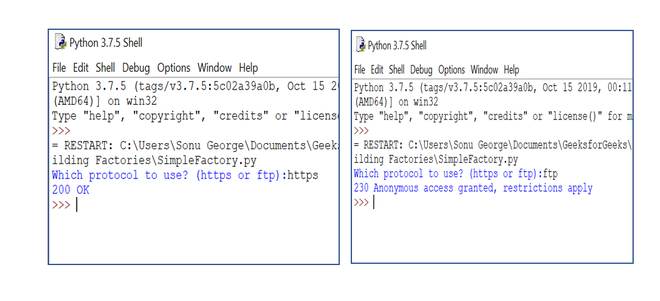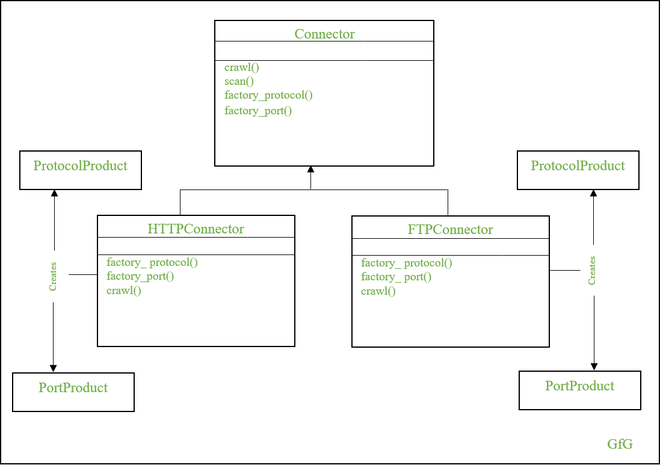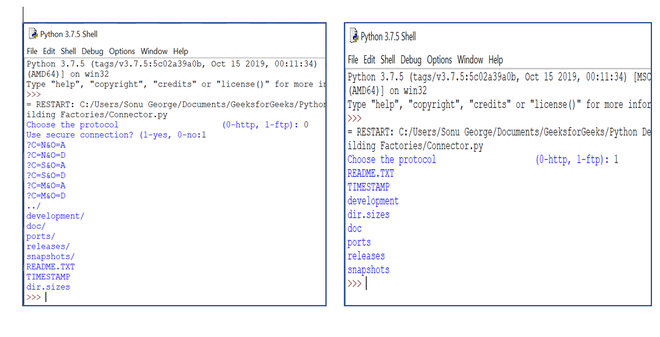# Accessing Web Resources using Factory Method Design Pattern in Python

• Last Updated : 01 Nov, 2020

A factory is a class for building other objects, where it creates and returns an object based on the passed parameters. Here, the client provides materials to the Factory class, and the Factory class creates and returns the products based on the given material. A Factory is not a design pattern, but it serves as a basis for the Factory Method Design Pattern.

Before getting into the Factory Method design pattern, let’s create a Simple Factory. In the below example, the SimpleFactory class has a static method called build_connection() to create objects based on the received parameter.

## Python3

 `import` `http.client``from` `ftplib ``import` `FTP`` ` ` ` `class` `SimpleFactory(``object``):``   ` `    ``@staticmethod``    ``def` `build_connection(protocol):``        ``if` `protocol ``=``=` `'https'``:``            ``return` `http.client.HTTPSConnection(``'www.python.org'``)``        ``elif` `protocol ``=``=` `'ftp'``:``            ``return` `FTP(``'ftp1.at.proftpd.org'``)``        ``else``:``            ``raise` `RuntimeError(``'Unknown protocol'``)`` ` ` ` `if` `__name__ ``=``=` `'__main__'``:``   ` `    ``input_protocol ``=` `input``(``'Which protocol to use? (https or ftp):'``)``    ``protocol ``=` `SimpleFactory.build_connection(input_protocol)``     ` `    ``if` `input_protocol ``=``=` `'https'``:``        ``protocol.request(``"GET"``, ``"/"``)``        ``resp ``=` `protocol.getresponse()``        ``print``(resp.status, resp.reason)``     ` `    ``elif` `input_protocol ``=``=` `'ftp'``:``        ``resp ``=` `protocol.login()``        ``print``(resp)`

Let’s look into the outputOutput

Here, you provide the type of protocol as an argument, and the factory class creates and returns the object based on the argument. The point to note is that object creation is not the responsibility of the client.

## Factory Method Design Pattern

Factory Method Design Pattern is identical to a simple factory, but its structure is complicated compared to a simple factory structure. It has an abstract class that contains factory methods (to create objects) and operation methods (to work with created objects).  And, the concrete classes that create objects are derived from the abstract class.

This pattern helps to define an interface to create an object. Here the classes that implement the interface decide which class to instantiate. Hence, the operation methods in the abstract class need not worry about object creation until the product interface is implemented.

Note: If you are a beginner, I strongly recommend you to go through the Factory Method – Python Design Patterns.

### Advantages of the Factory Method Design Pattern

Let’s look into the advantages of the Factory Design Pattern. A few of them are:

1. It makes code more universal
2. It separates interfaces from implementation
3. It reduces the complexity of code maintenance.

### Accessing Web Resources Using Different Protocol

Let’s implement a program for accessing web resources using HTTP or FTP protocol. Here, we will use the site ftp.freebsd.org that allows both HTTP and FTP protocol.Factory Method Design Pattern

From the above diagram, we can understand that the design has an abstract class called Connector, and two concrete classes – HTTPConnector and FTPConnector. The two concrete classes are derived from the Connector class, and the factory methods in the abstract class use these classes for product creation. Let’s look into the code below.

## Python3

 `import` `abc``import` `urllib``import` `urllib.error``import` `urllib.request``from` `bs4 ``import` `BeautifulSoup`` ` ` ` `class` `Connector(metaclass``=``abc.ABCMeta):``    ``def` `__init__(``self``, is_secure):``        ``self``.is_secure ``=` `is_secure``        ``self``.port ``=` `self``.factory_port()``        ``self``.protocol ``=` `self``.factory_protocol()`` ` `    ``@abc``.abstractmethod``    ``def` `crawl(``self``):``        ``pass`` ` `    ``def` `scan(``self``, con_domain, con_path):``        ``url ``=` `self``.protocol ``+` `'://'``+` `con_domain \``              ``+` `':'` `+` `str``(``self``.port) ``+` `con_path``        ``print``(url)``        ``return` `urllib.request.urlopen(url, timeout``=``10``).read()`` ` `    ``@abc``.abstractmethod``    ``def` `factory_protocol(``self``):``        ``pass`` ` `    ``@abc``.abstractmethod``    ``def` `factory_port(``self``):``        ``pass`` ` `class` `HTTPConnector(Connector):``    ``""" Creates an HTTP Connector """`` ` `    ``def` `factory_protocol(``self``):``        ``if` `self``.is_secure:``            ``return` `'https'``        ``return` `'http'`` ` `    ``def` `factory_port(``self``):``        ``if` `self``.is_secure:``            ``return` `'443'``        ``return` `'80'`` ` `    ``def` `crawl(``self``, data):``        ``""" crawls web content """``        ``filenames ``=` `[]``        ``soup ``=` `BeautifulSoup(data,``"html.parser"``)``        ``links ``=` `soup.table.findAll(``'a'``)``         ` `        ``for` `link ``in` `links:``            ``filenames.append(link[``'href'``])``        ``return` `'\n'``.join(filenames)`` ` `class` `FTPConnector(Connector):``    ``def` `factory_protocol(``self``):``        ``return` `'ftp'`` ` `    ``def` `factory_port(``self``):``        ``return` `'21'`` ` `    ``def` `crawl(``self``, data):``        ``# converting byte to string``        ``data ``=` `str``(data, ``'utf-8'``)``        ``lines ``=` `data.split(``'\n'``)``        ``filenames ``=` `[]``        ``for` `line ``in` `lines:``            ``extract_line ``=` `line.split(``None``, ``8``)``            ``if` `len``(extract_line) ``=``=` `9``:``                ``filenames.append(extract_line[``-``1``])``                 ` `        ``return` `'\n'``.join(filenames)`` ` `if` `__name__ ``=``=` `"__main__"``:``    ``con_domain ``=` `'ftp.freebsd.org'``    ``con_path ``=` `'/pub/FreeBSD/'`` ` `    ``con_protocol ``=` `input``('Choose the protocol \``                    ``(``0``-``http, ``1``-``ftp): ')``     ` `    ``if` `con_protocol ``=``=` `'0'``:``        ``is_secure ``=` `input``(``'Use secure connection? (1-yes, 0-no):'``)``        ``if` `is_secure ``=``=` `'1'``:``            ``is_secure ``=` `True``        ``else``:``            ``is_secure ``=` `False``        ``connector ``=` `HTTPConnector(is_secure)``    ``else``:``        ``is_secure ``=` `False``        ``connector ``=` `FTPConnector(is_secure)`` ` `    ``try``:``        ``data ``=` `connector.scan(con_domain, con_path)``    ``except` `urllib.error.URLError as e:``        ``print``(``'Cannot access resource with this method'``, e)``    ``else``:``        ``print``(connector.crawl(data))``        `

In this program, we connect to a web page using the HTTP or FTP protocol. The Connector abstract class is designed to establish a connection (scan method), and as well as for crawling the pages (crawl method). In addition to this,  it provides two factory methods – factory_protocol and factory_port – to deal with protocol and port address.

Let’s look into the output.Output

## Summary

A factory class is used to create other classes, It makes the code more universal. The factory methods in factory classes act as an interface for creating an object, thereby it separates interface from implementation. As a result, classes can implement these interface methods and can decide which class to instantiate.

My Personal Notes arrow_drop_up